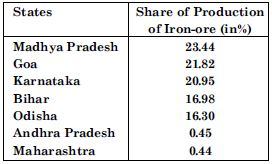# NCERT Solutions for Class 12 Geography Chapter 4 - Use of Computer in Data Processing and Mapping

##### Question 1:

What type of graph would you use to represent the following data?1. Line
2. Multiple Bar Graph
3. Pie Diagram
4. None of the above

(c) Pie Diagram

##### Question 2:

Districts within a state would be represented in which type of spatial data?

1. Points
2. Lines
3. Polygons
4. None of the above

(a) Points

##### Question 3:

Which is the operator that is calculated first in a formula given in a cell of a worksheet?

1. +
2. -
3. /
4. x

(d) x

##### Question 4:

Function wizard in Excel enables you to

1. Construct graphs
2. Carryout mathematical/statistical operations
3. Draw maps
4. None of the above

(a) Construct graphs

##### Question 5:

What are the functions of different parts of a computer?

A computer is very fast and versatile machine. This machine can perform simple arithmetic operations such as addition, subtraction, division and multiplication and this can also solve mathematical formulas. It can be used for data processing, preparation of diagrams, graphs and maps etc.

##### Question 6:

What are the advantages of using computer over manual methods of data processing and representation?

Computer saves times and improve efficiency in data processing and graphical presentation. Computer is more versatile as it facilitates the on screen editing the text copy and move it from one place to another or even delete the unwanted material. It can handle a large volume of the data and it further enables, validation, checking and correction etc.

##### Question 7:

What is a worksheet?

MS Excel is also called spread sheet. It is the work-sheet. The spread-sheets are located in workbooks or excel files. Excel worksheet contains 16384 rows and 256 columns.

##### Question 8:

What is differences between spatial and non-spatial data? Explain with examples.